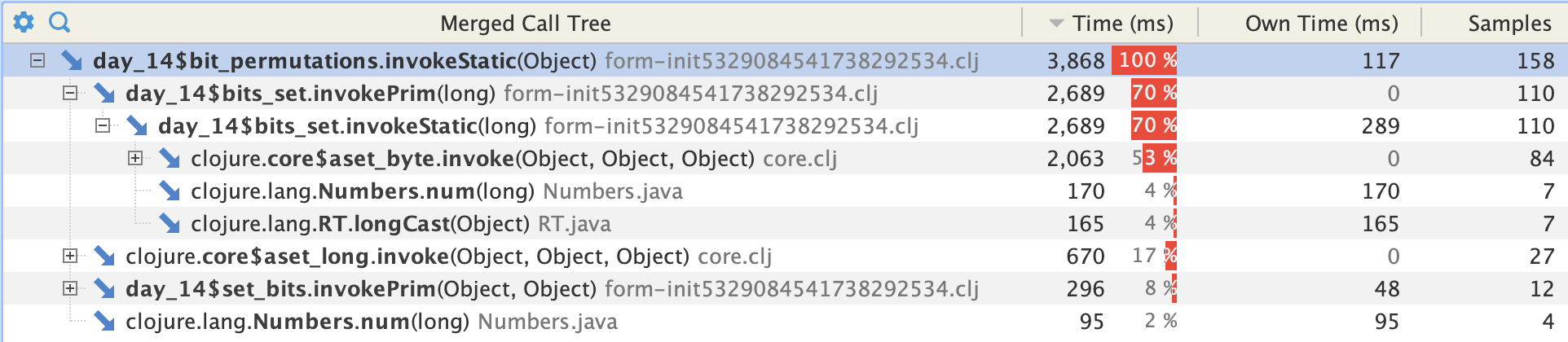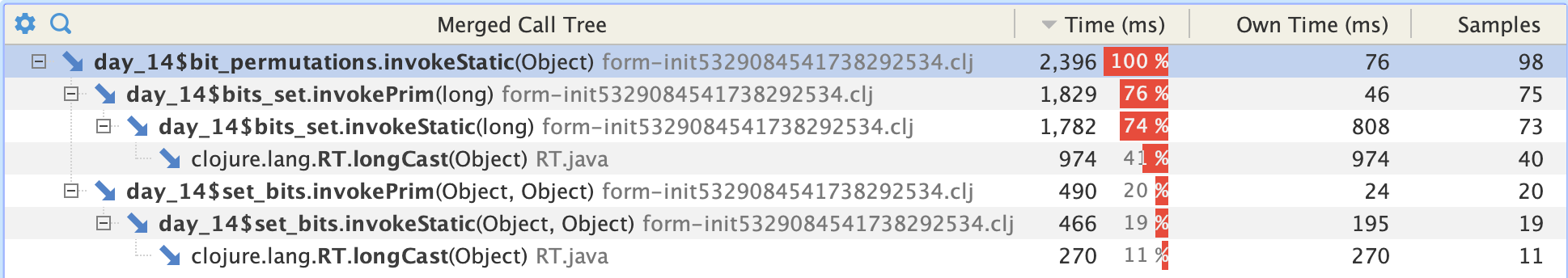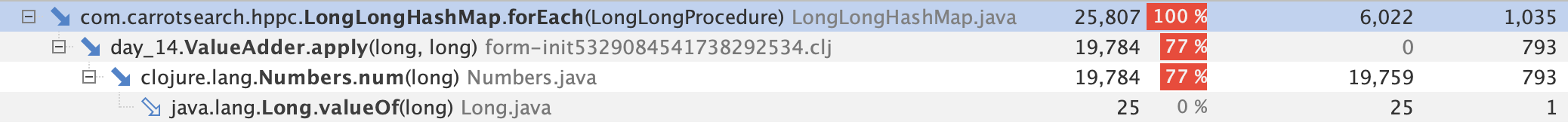# aset-byte considered harmful

I've been making a bit of a meal of Advent of Code this year, using Clojure to polish up my skills rather than attempting to expand them. As such I don't really mind that I've spent a couple of days finding newer and closer ways to shave a particular yak. As the shave became closer and closer I discovered a new thing about aget-type vs aget, so it wasn't a total waste of time:-)

Day 14 Part 2 in the end is a comparative doddle. I have 559 lines of input which break down into 100 mask assignments and 459 pokes into a 36-bit memory address space. Unlike Part 1, an "X" in the mask implies a fuzzy bit that could be 1 or 0. Therefore any given memory write needs to be exploded out across a number of addresses. The question in the end is the sum of the memory contents.

The clever way to do this would keep track of the masks and writes. A write to an XX mask yields 2^2 permutations hence 4x the write in terms of the end sum. Of course you need to deal with overlapping writes, so it's not as easy to do as it is to conceptualise, so I just did it via brute force which was sufficient:-)

Rather than create a full 36-bit memory arena which might be slightly expensive, I use a map of address to value. The most permuted mask has 2^9 open combinations so my worst case is about 235K memory locations to track.

Enough preamble and onto the yak shaving. The original idiomatic clojure implementation is here and the latest and greatest here. There are two main areas where the natural approach can be improved on from a performance perspective. 80ms vs 5ms would be a worthwhile speedup if this wasn't code that gets executed once:-)

1. Data structure for the memory arena.
2. Bit permutation.

## 1. Data structure of the memory arena.

This is dangerously close to home territory for me, dealing with "lots" of data in constrained processing environments. The Clojure datastructures are immutable for good reasons, but a core of aggregation and merge technologies can demand a bit more of a low-level approach. Note that I'm only throwing one thread at this problem anyway, because the answer is fairly path dependent (I guess I could generate snapshots of the memory arena and then merge them in parallel, but it feels like the merge step might be as expensive as solving the problem).

Anyhoo, given we're in the JVM, it was comparatively easy for me to drop in the carrotsearch hppc library and make use of their LongLongHashMap. I could have tried an intermediate approach with java.util.HashMap or similar, it might be interesting to see how much of the benefit is gained even when you still have to box, but happy to leave that as an EEIR.

```(ns day-14
(:require [clojure.string :refer [split split-lines replace]])
(:use clojure.test)
(:import [com.carrotsearch.hppc LongLongHashMap]
[com.carrotsearch.hppc.procedures LongLongProcedure]))```

Writes into the map are straightforward, same value to up to up to 2^9 addresses at once. areduce is great, no need for loop-recur shenanigans even though we've gone primitive.

```(defn turbo-assign [^LongLongHashMap memory ^longs addresses ^long value]
(areduce addresses i ret (int 0) (.put memory (aget addresses i) value))
memory)```

The LongLongProcedure interface allows us to sum the memory arena with .forEach, this shows how easy Java interop can be. The horrors of mutable state though!

```(defprotocol ValueRetriever
(get-value [this ^LongLongHashMap memory]))

(deftype ValueAdder [^{:unsynchronized-mutable true} ^long total]
LongLongProcedure
(^void apply [this ^long k ^long v] (set! total (+ total v)))
ValueRetriever
(get-value [this memory] (set! total 0) (.forEach memory this) total))```

## 2. Bit permutation.

At this point I was down from 80ms runtime to 13ms runtime. After all this effort it's probably worth checking for any easy adjustments we can make.So, given a mask of bits which are both 0 and 1, how do we generate all the valid bit permutations in a speedy manner? My approach is pretty straightforward - what bits are set in my mask? How many are they? Create an array therefore big enough to hold them, then for each index take which bits are set and map it onto our set of bits.

```(defn bit-permutations
"Return an array of longs which are all the possible permutations of the bits in the input long."
([l]
(let [^bytes our-bits (bits-set l)
how-many-bits (alength our-bits)
n-permutations (Math/pow 2 how-many-bits)
^longs permutations (long-array n-permutations)]
(areduce ^longs permutations i ret (long 0)
(long
(aset-long ^longs permutations i (set-bits our-bits (bits-set i)))))
permutations)))```

bits-set is at the core of our performance. this returns an array with the index of the bits that are set.

```(defn bits-set ^bytes [^long x]
"Return which bits are set in a given long"
(let [len (Long/bitCount x)]
(loop [l x s (byte-array len) i (int 0) b (byte 0)]
(if (= i len) s
(let [is-set (when (= 1 (bit-and 1 l)) (aset-byte s i b))]
(recur (bit-shift-right l 1) s (if is-set (inc i) i) (byte (inc b))))))))```

Quite a lot of function, but why are we spending half our time setting a byte into an array? That really is not where I'm expecting the critical path to be.

After some experimentation, I find that the untyped "aset" performs much much faster than "aset-bytes"

```day-14> (defn aset-byte-test []
(let [arr (byte-array 100000)]
(areduce arr i ret 0 (aset-byte arr i 23))))
#'day-14/aset-byte-test
day-14> (criterium.core/quick-bench (aset-byte-test))
Evaluation count : 96 in 6 samples of 16 calls.
Execution time mean : 6.613403 ms
nil
day-14> (defn aset-test []
(let [arr (byte-array 100000) b (byte 23)]
(areduce ^bytes arr i ret (byte 0) (aset arr i b))))
#'day-14/aset-test
day-14> (criterium.core/quick-bench (aset-test))
Evaluation count : 2094 in 6 samples of 349 calls.
Execution time mean : 287.939166 µs
nil
day-14> (defn aset-byte-test []
(let [arr (byte-array 100000) b (byte 23)]
(areduce arr i ret (byte 0) (aset-byte arr i b))))
Syntax error (IllegalArgumentException) compiling fn* at (*cider-repl dev/advent-2020:localhost:60264(clj)*:25:9).
recur arg for primitive local: ret is not matching primitive, had: Object, needed: byte```

The last attempt is my failure to "primitive-ify" the calls to aset-byte. It seems that it works in boxed Objects, which might explain some of the performance problems. Switching from aset-byte and aset-long to just aset moves the dial on performance substantially,## Conclusions

Let's have a look at the final timings

TimeTest
79.8msIdiomatic clojure
13.1msSame algorithm, primitive arrays, aset-bytes and aset-long
5.9msJust using aset
5.2msStop casting to long in set-bits

Of course, to answer the exam question I needed to run this code just once. Unnecessary optimisation is the root of all learning?

tl;dr use aset, never aset-type; if you need a fast core, go primitive

## Postscript

With everything else tuned, the code to sum the arena is half the work - and 100% of it (based on samples) is just a pointless box. There's always the next layer of yak to shave.## Credits

Indispensible tools: Criterium for benchmarking, YourKit for profiling, and my new friend clj-java-decompiler